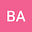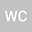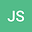On some connection results between Laguerre polynomials via third-order differential operator
•••• wathek chammam,
Universite de Gabes
Author Profilewathek chammam
Universite de Gabes
Author ProfileLet $\{L^{(\alpha)}_n\}_{n\geq 0}$, ($\alpha\neq-m, \ m\geq1$), be the monic orthogonal sequence of Laguerre polynomials. We give a new differential operator, denoted here $\mathscr{L}^{+}_{\alpha}$, raises the degree and also the parameter of $L^{(\alpha)}_n(x)$. More precisely, $\mathscr{L}^{+}_{\alpha}L^{(\alpha)}_n(x)=L^{(\alpha+1)}_{n+1}(x), \ n\geq0$. As an illustration, we give some properties related to this operator and some other operators in the literature, then we give some connection results between Laguerre polynomials via this new operator.